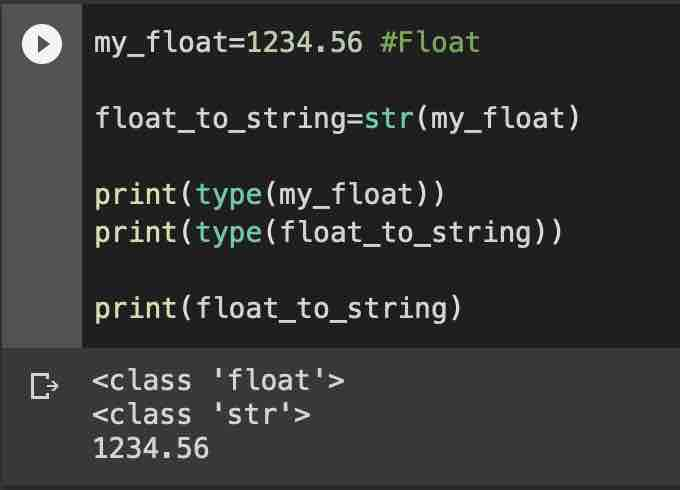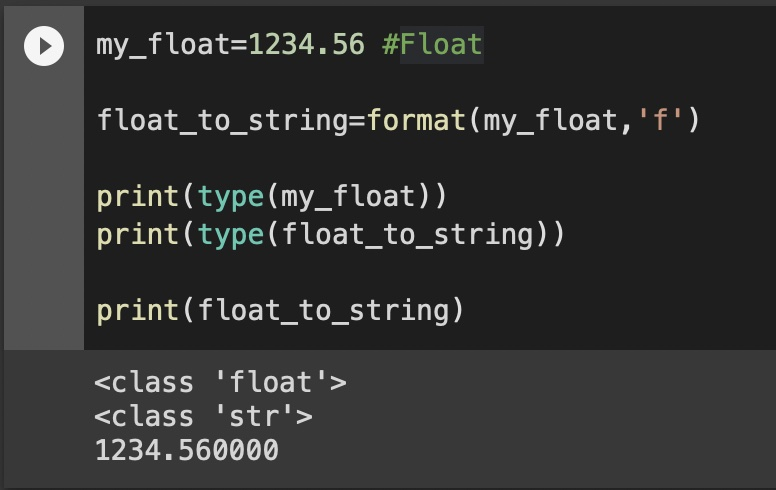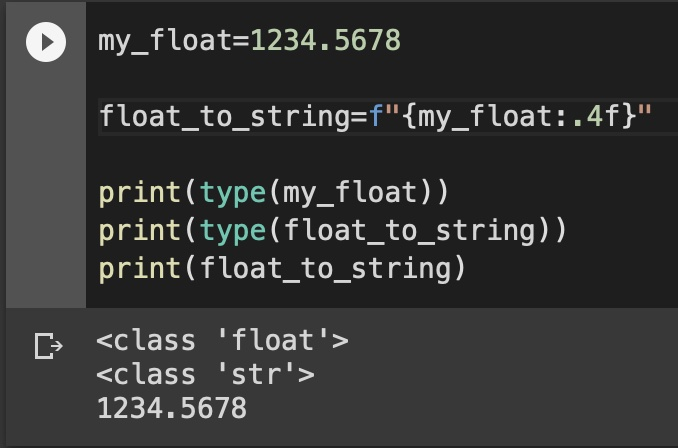# Python - Convert float to String

## Example 1: Using str() method

``````my_float=1234.56 #Float

float_to_string=str(my_float)

print(type(my_float))
print(type(float_to_string))

print(float_to_string)``````
Output:
``````<class 'float'>
<class 'str'>
1234.56``````## Example 2: using format() method

``````my_float=1234.56 #Float

float_to_string=format(my_float,'f')

print(type(my_float))
print(type(float_to_string))

print(float_to_string)``````
Output:
``````<class 'float'>
<class 'str'>
1234.560000``````## Example 3: Using format string

``````my_float=1234.5678

float_to_string=f"{my_float:.4f}"

print(type(my_float))
print(type(float_to_string))
print(float_to_string)``````
Output:
``````<class 'float'>
<class 'str'>
1234.5678``````-

### Facing issues? Have Questions? Post them here! I am happy to answer!Author Info:

Rakesh is a seasoned developer with over 10 years of experience in web and app development, and a deep knowledge of operating systems. Author of insightful How-To articles for Code2care.